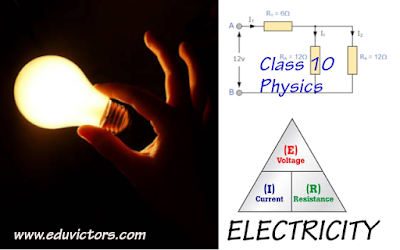## CHAPTER-12 ELECTRICITY

VERY SHORT ANSWER QUESTIONS
CARRYING 1 MARK WITH ANSWER

Q1: What is represented by joule/coulomb?

Answer: It represents potential difference.

Q2: A charge of 2C moves between two plates, maintained at a p.d of 1V. What is the energy acquired by the charge?

Q3: Why are copper wires used as connecting wires?

Answer: The electrical resistivity of copper is low

Q4: A wire of resistivity 10 ohm meter is stretched to double its length. What is its new resistivity?

Answer: It remains same because resistivity depends on nature of material.

Q5: What is the resistance of connecting wire?

Answer: The resistance of a connecting wire, which is made of good conductor, is negligible.

Q6: What should be the resistance of an ammeter?

Answer: The resistance of an ammeter should be very small and for an ideal ammeter, its value is zero.

Q7: What should be the resistance of a Voltmeter?

Answer: The resistance of a voltmeter should be  very high and for an ideal voltmeter, its value is infinity.

Q8: Which has more resistance: 100W bulb or 60W bulb?

Answer: As R is inversely proportional to P for constant V. Thus, the resistance of 60W bulb is more.

Q9: How will you join three resistances, each of 2 ohm so that the effective resistance is 3 ohm?

Answer: A parallel combination of two resistances (which will be 1 ohm) joined in series with the third resistance (2 ohm)

Q10: What happens to the current in a circuit if its resistance is doubled?

Answer: As I inversely proportional to R, the current is reduced to half of its previous value.

Q11: What happens to the resistance of a circuit if the current through it is doubled?

Answer: The resistance of the circuit does not depend on the current through it, it will remain the same.

Q12: How does the resistance of a wire depend upon its radius?

Answer: As R is  inversely proportional to A, R is inversely proportional to square of radius r.

Q13: Two wires are of the same length, same radius, but one of them is of copper and the other is of iron. Which will have more resistance?

Answer: A and l are same R depends only on resistivity and it is more for iron so iron has more resistance.

Q14: Two wires of same material and same length have radii r1 and r2. Compare their resistances.

Answer: R inversely proportional to square of radius r.
R1/R2 =(r2/r1)²

Q.15 Given n resistors each of resistors Rs. How will you combine them to get the
(i) maximum and
(ii) minimum effective resistance?
What is the ratio of the maximum to minimum resistance?

Answer: For maximum resistance Rs= nr (Equivalent of series combination)
For minimum resistance Rp= r/n (Equivalent of parallel combination)
Rs/Rp= n2

Ch 12 - Electricity  (Q & A, NCERT Solutions and Numerical)
Ch 12 - Electricity (MCQs)
Ch 12 - Electricity (Worksheet)
Ch 12 - Electricity (Important Points To Remember)
Ch 12 - Electricity (Holiday Assignment)
Ch 12 - Electricity (Assignment)